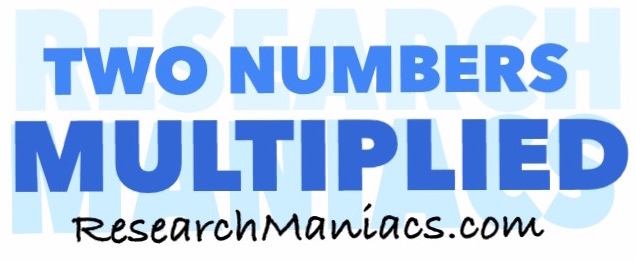What two numbers multiply to 3?What 2 numbers do you multiply to get 3? In other words, what number can you multiply with another number to get 3?

The formula to solve this would be:

X x Y = 3

There are at least two combinations of two numbers that you can multiply together to get 3.

For your convenience, we have made a list of all the combinations of two numbers multiplied by each other that will make 3:

1 x 3 = 3
3 x 1 = 3

Two Numbers Multiplied
Enter another number below to see which combinations of two numbers multiplied will equal that number.

What two numbers multiply to 4
Go here for the next number on our list.